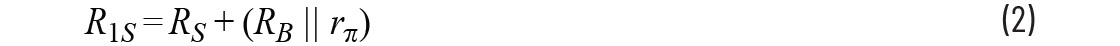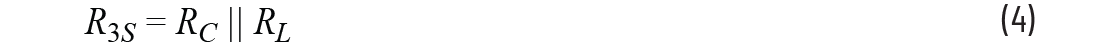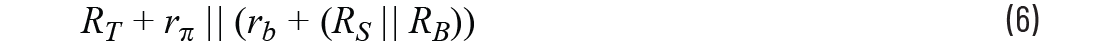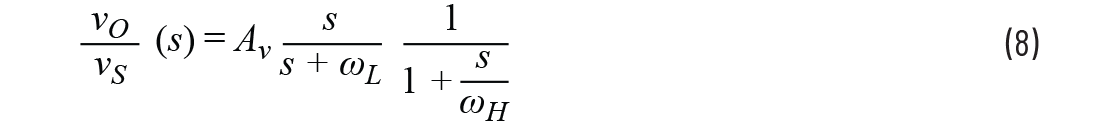# ADALM2000 Activity: Frequency Response of a Common-Emitter BJT Amplifier

### Objective:

The objective of this activity is to investigate the frequency response of the common emitter amplifier configuration using an NPN BJT transistor.

### Common Emitter Amplifier Topology

The schematic of a typical common emitter amplifier is shown in Figure 1. Capacitors CB and CC are used to block the amplifier dc bias point from the input and output (ac coupling). Capacitor CE is an ac bypass capacitor used to establish a low frequency ac ground at the emitter of Q1. Miller capacitor CF is a small capacitance that will be used to control the high frequency 3 dB response of the amplifier.

### Low Frequency Response

Figure 2 shows the low frequency, small signal equivalent circuit of the amplifier. Note that CF is ignored since it is assumed that its impedance at these frequencies is very high. RB is the parallel combination of RB1 and RB2.

Using short-circuit time constant analysis, the lower 3 dB frequency (ωL) can be found as:Where### High Frequency Response

Figure 3 shows the high frequency, small signal equivalent circuit of the amplifier. At high frequencies, CB, CC, and CE can be replaced with short circuits since their impedance becomes very small compared to RS, RL, and RE.

The higher 3 dB frequency (ωH) can be derived as:WhereThus, if we assume that the common emitter amplifier is properly characterized by these dominant low and high frequency poles, then the frequency response of the amplifier can be approximated by:Where:

s is the complex angular frequency

AV is the midband gain

ωL is the low corner angular frequency

ωH is the high corner angular frequency

#### Pre-Lab Setup

Assuming CB = CC = CE = 1 farad and CF = CΠ = Cμ = 0, and, using a 2N3904 transistor, design a common emitter amplifier with the following specifications:

VCC = 5 V
RS = 50 Ω
RL = 1 kΩ
RIN >250 Ω
ISUPPLY <8 mA
AV >50
Peak-to-peak unclipped output swing >3 V

• Show all your calculations, design procedure, and final component values.
• Verify your results using the LTspice® circuit simulator. Submit all necessary simulation plots showing that the specifications are satisfied. Also provide the circuit schematic with dc bias points annotated.
• Using LTspice, find the higher 3 dB frequency (fH) while CF = 0.
• Determine Cπ, Cμ, and rb of the transistor from the simulated operating point data. Calculate fH using the equation from the High Frequency Response section and compare it with the simulation result obtained in Step 3. Remember that the equation gives you the radian frequency and you need to convert to Hz.
• Calculate the value of CF to have fH = 5 kHz. Simulate the circuit to verify your result and adjust the value of CF if necessary.
• Calculate CB, CC, CE to have fL = 500 Hz. Simulate the circuit to verify your result and adjust the values of capacitors if necessary.

### Lab Procedure

#### Objective:

The objective of this section of the lab activity is to validate your pre-lab design values by building the actual circuit and measuring its frequency response performance.

#### Materials:

• Six resistors, various values, from the ADALP2000 analog parts kit
• Four capacitors, various values, from the ADALP2000 analog parts kit
• One small signal NPN transistor (2N3904)

Note that on the source resistor, RS, and the AWG output of the ADALM2000, the AWG output has a 50 Ω series output resistance and you will need to include it, along with the external resistance, in series with its output. Also, due to the relatively high gain of your design, you will need an input signal with a small amplitude of around 100 mV peak-to-peak. Rather than turning down the AWG in software, it would be better from a noise point of view to insert a resistor voltage divider between the AWG output and your circuit input to attenuate the signal. Using something like the setup shown in Figure 4 will provide both an attenuation factor of 1/16 and a 60 Ω equivalent source resistance. Other combinations of resistor values are also possible based on what you have available—in our case, a standard resistor value will be used—68 Ω.Figure 4. A signal attenuator with a 68 Ω source resistance.

#### Directions

• Construct the amplifier, based on the schematic in Figure 1, you designed in the pre-lab. Based on your design values from the pre-lab, use the closest standard value from your kit. Remember that you can combine the standard values in series or parallel to get a combined value closer to your design number.
• Check your dc operating point by measuring IC, VE, VC, and VB. If any dc bias value is significantly different than the one obtained from simulation, modify your circuit to get the desired dc bias before moving onto the next step.
• Measure ISUPPLY.
• Use the network analyzer instrument in the Scopy software to obtain the magnitude of the frequency response of the amplifier from 50 Hz to 20 kHz and determine the lower and upper 3 dB frequencies fL and fH.
• At midband frequencies, measure AV, RIN, and ROUT.

Plot examples are provided using the LTspice simulations of the circuit in Figure 5.Figure 7. LTspice ac sweep plot with CF = 0.047 μF.Figure 8. LTspice plot with CF = 0.047 μF at frequency = 1.5 kHz.

### Question:

• Replace capacitor CF with a smaller value (0.01 μF) and remeasure the response curve with the network analyzer instrument or ac sweep simulation. Explain the effect of the new capacitor value in the response that you see.

You can find the answer at the StudentZone blog.